## What is Algebra and Algebraic Expressions?

Practice Unlimited Questions

#### 1. What is algebra?

Algebra is the branch of mathematics in which we represent unknown numbers or quantities in terms of letters or symbols.

The unknown number is also called a variable.

#### 2. How do we write algebraic expressions?

Example: At any time, Pinky had \$7 more than Greene.
Let's learn how to write this statement as an algebraic expression.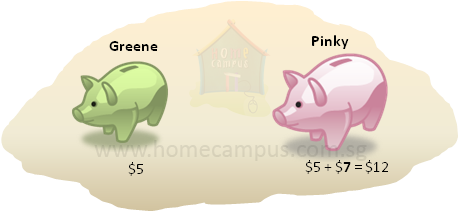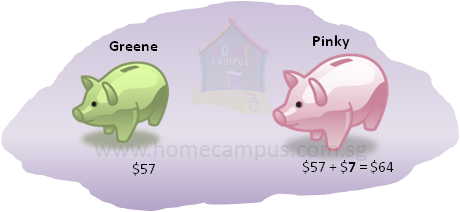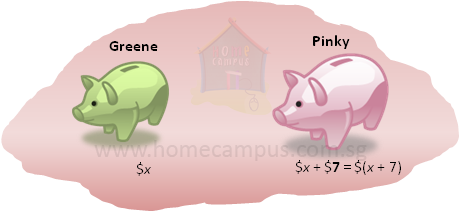So, Pinky had \$(x + 7).
We use a letter to represent the unknown number.
x is the unknown number here.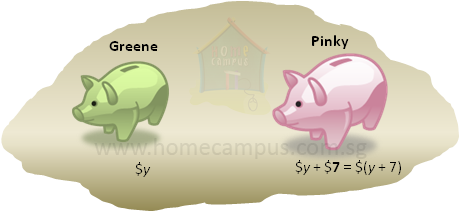We can use any letter to represent an unknown number.
We use the letter y to represent the unknown number here.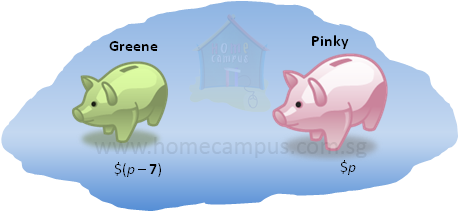So, Greene had \$(p  −  7).

Since Pinky had \$7 more than Greene, this means Greene had \$7 less than Pinky.
Let's put the above in a table.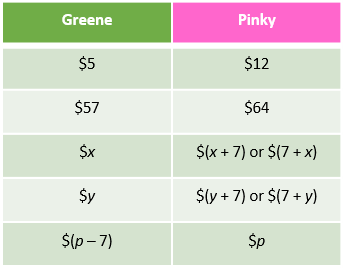(x + 7), (7 + x), (y + 7), (7 + y) and (p - 7) are all examples of algebraic expressions.

We say that,
• (x + 7) and (7 + x) are algebraic expressions in terms of x.
• (y + 7) and (7 + y) are algebraic expressions in terms of y.
• (p - 7) is an algebraic expression in terms of p.

#### 3. Section A has more kids than Section B. There are 37 kids in Section A and x kids in Section B. a)  How many kids are there in Section A and Section B together in terms of x? b)  How many more kids are there in Section A than in Section B expressed in terms of x?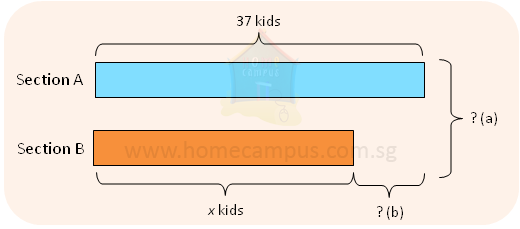a)  Number of kids in Section A  =  37
Number of kids in Section B  =  x
Number of kids in Section A and B together  =  37 + x
b)  Number of kids in Section A  =  37
Number of kids in Section B  =  x
The difference between the number of kids in Sections A and B  =  37  −  x

There are (37  −  x) more kids in Section A than in Section B.

#### 4. There are 7 marbles in a bag. a)  How many marbles are there in 3 bags? b)  How many marbles are there in k bags? Write the algebraic expression in terms of k.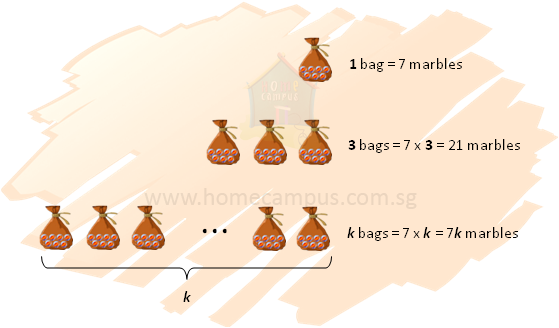There are 7 marbles in 1 bag.

a)  There are 7  ×  3 or 21 marbles in 3 bags.
b)  There are 7  ×  k or 7k marbles in k bags.
We write 7  ×  k as 7k.
Also, k  ×  7 = 7k

#### 5. Sarah had t rolls of ribbon. She kept 2 rolls for herself and distributed the rest equally among 3 of her cousins. How many rolls did each cousin get? Express the answer in terms of t.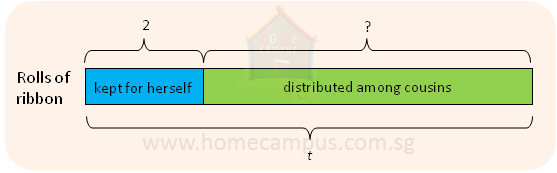Total number of rolls that Sarah had at first  =  t
Number of rolls that Sarah kept for herself  =  2
Total number of rolls that Sarah distributed among the cousins  =  (t  −  2)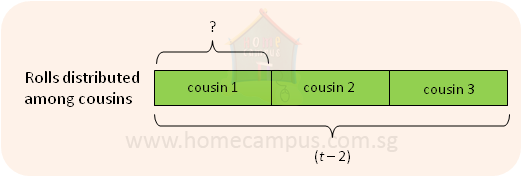Number of cousins  =  3
Number of rolls each cousin got  =  (t  −  2)  ÷  3  =
 t - 2 3

We write (t  −  2) ÷ 3 as
 (t - 2) 3
or
 1 3
× (t  −  2).